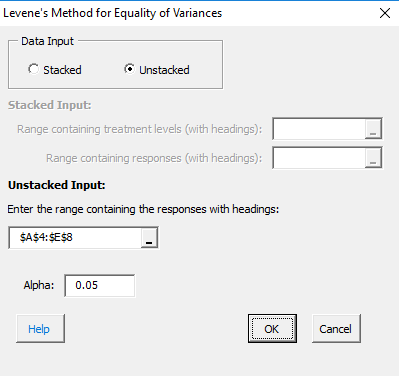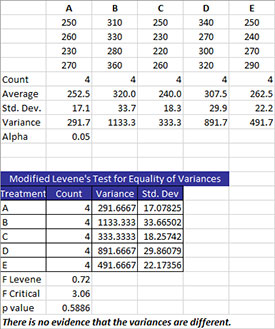# Modified Levene’s Test for Equality of Variances HelpThe Modified Levene’s Test is used to determine if the variances from multiple treatments (or processes) are the same.

There are two options for entering the data: stacked or unstacked. Stacked data have each treatment in a single column. Unstacked data have the treatment labels in one column and the results in the adjacent column. Please refer to the Stacked and Unstacked Data Entry section for more information. The example below uses unstacked data.

Five different treatments (A to E) were used to control the weight of a coating in grams. There were four samples for each treatment. We want to use Bartlett’s test method to determine if there are any significant differences in the treatment variances.

1. Enter the data as shown below. The column headings represent the treatment labels with the responses for each treatment under the label. The number of results for each treatment do not have to be equal. The data can be downloaded at this link.2. Select the data and the column headings.

3. Select “Multiple Processes” from the “Statistical Tools” panel in the SPC for Excel ribbon.

4. Select the “Modified Levne’s Test for Equality of Variances” option. Select OK and the input form below is displayed.• Data Input: there are two options: stacked and unstacked. If the range selected on the worksheet contains more than two columns, the default is unstacked; this can be changed. For more information on stacked versus unstacked data, click here.
• Stacked Option: these are the ranges to be entered:
• Range containing the treatment levels.
• Range containing the responses.
• Unstacked Option:
• The data must be columns with the first cell in each column representing the treatment level.
• Alpha: this is the confidence level; 1-alpha is the confidence interval; default is 0.05 for 95% confidence.
• Select OK to generate the results.
• Select Cancel to end the program.

Modified Levene’s Method Test for Equality of Variances Output

The output from Levene’s Test is shown below.A new worksheet is added to the workbook. The top part of the worksheet contains the data in stacked format along with some statistics for each treatment including count, average, standard deviation, and variance. The value of alpha is also given. The bottom part of the worksheet contains the information from Levene’s Method. The treatments are listed along with the variance and standard deviation. The following three statistics are given:

• F Levene: This is the Levene statistic given by (where N = total number of observations and a = number of treatments):• F Critical: This the critical value of F based on alpha with a-1 degrees of freedom in the numerator and N-t degrees of freedom in the denominator; reject the null hypothesis if F Levene > F Critical.
• p value: reject the null hypothesis if p value < alpha.